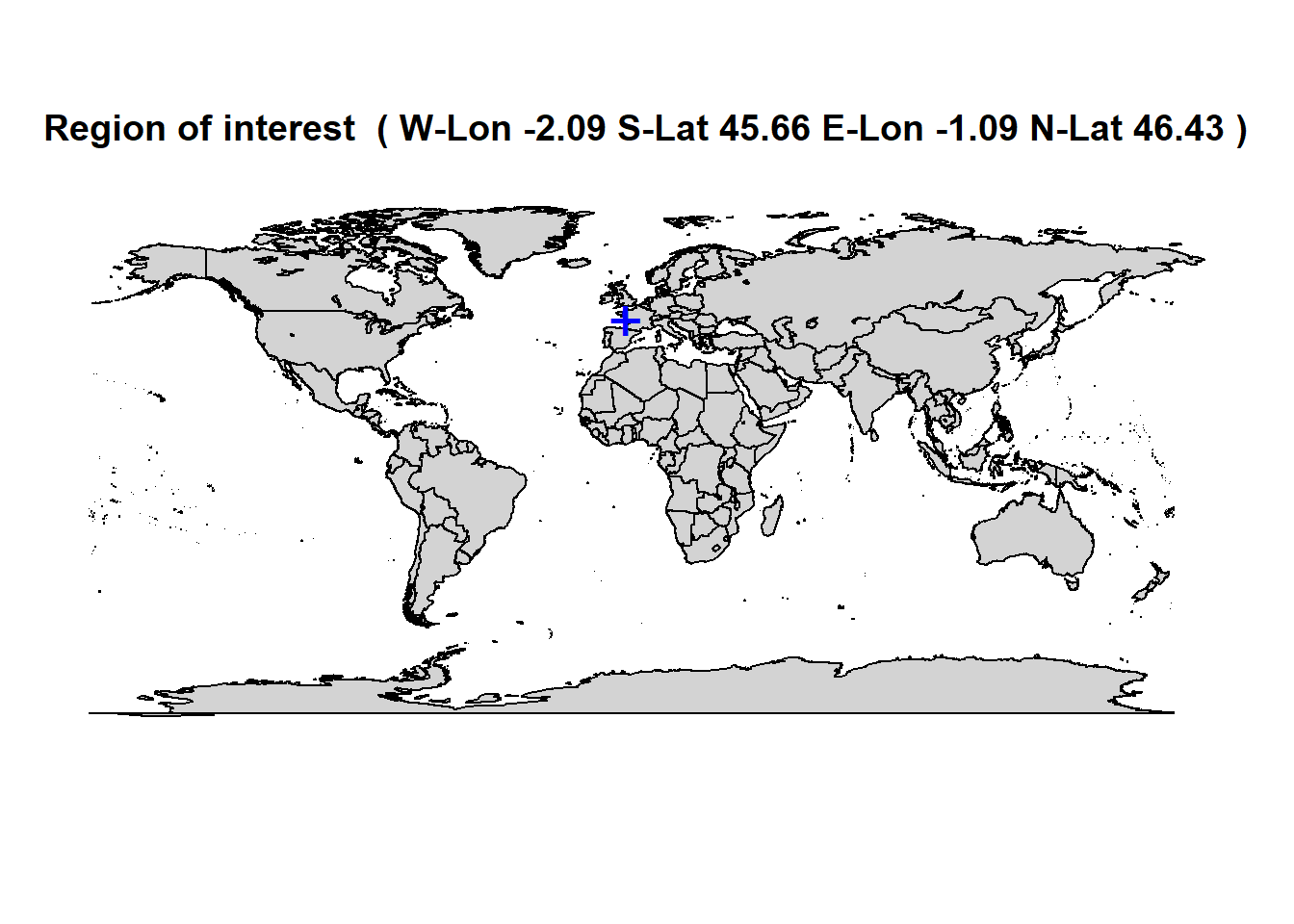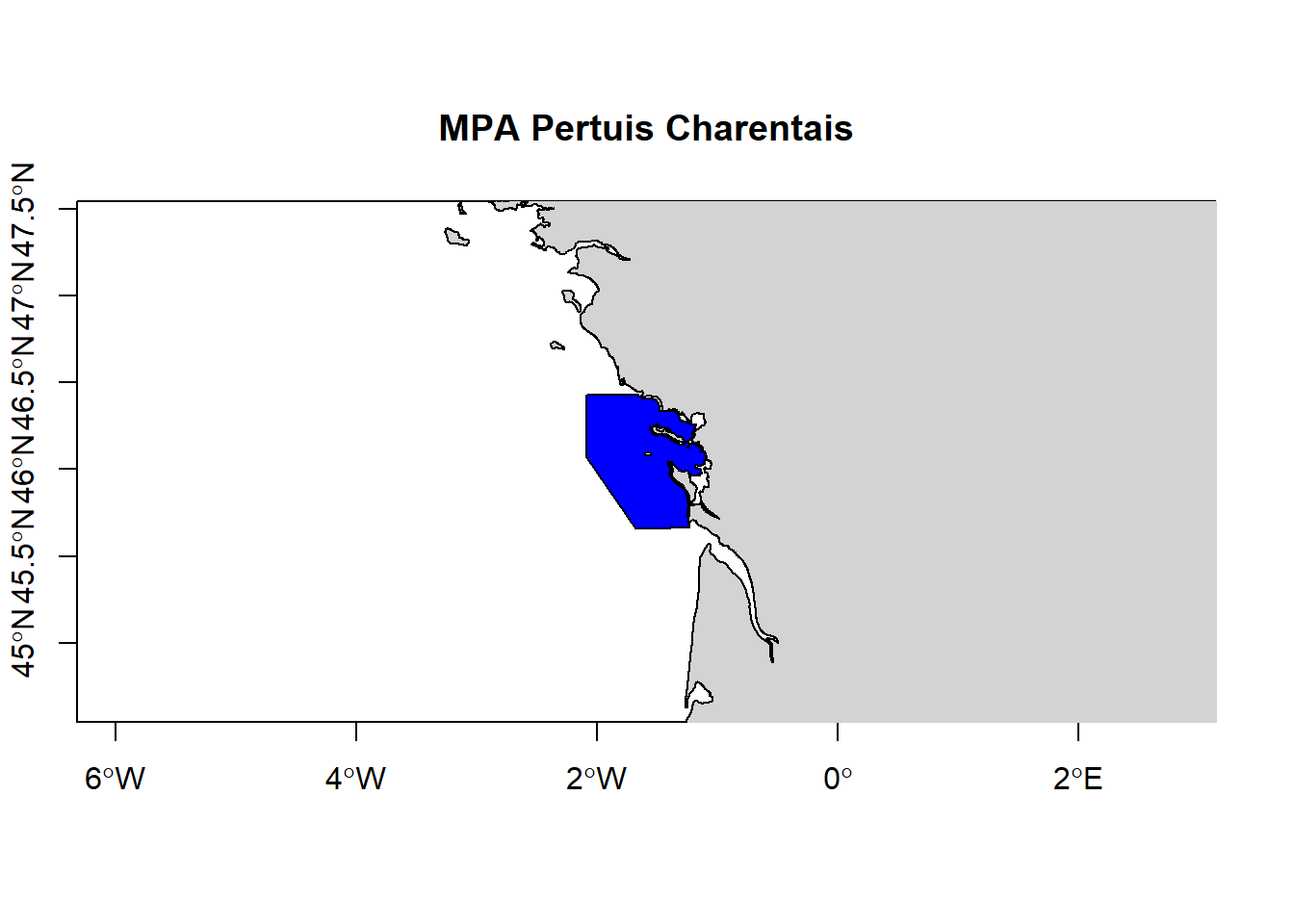We will use the R packages listed below. They can be downloaded using the `install.packages()` command. In case you are running R on Windows, you may need to download Rtools to build the packages.

``````# install.packages(c("dplyr","tidyr","wdpar","rgdal","rasterVis","downloader","directlabels","ggplot2","rgl","ncdf4","mapdata","maptools","geojsonio","lattice","reshape2","XML"))
library(dplyr)
library(tidyr)
library(wdpar)
library(rgdal)
library(rasterVis)
library(directlabels)
library(ggplot2)
library(rgl)
library(ncdf4)
library(mapdata)
library(maptools)
library(geojsonio)
library(lattice)
require(reshape2)
library(XML)``````

During the first four exercises, we focus on the Pertuis Charentais Marine Protected Area (MPA) in France. The shapefile for this area can be downloaded from the World Database on Protected Areas (WDPA). For the sake of this tutorial, we will download it with the dedicated WDPA R-package `wdpar`.

``````# get the MPA Shapefile from WDPA
wdpaid <- "555526224"
mpa <- as_Spatial(wdpa_fetch("France") %>% filter(WDPAID == wdpaid))
# mpa <- shapefile("path/to/WDPA_July2018_protected_area_555526224-shapefile-polygons.shp")

# get the spatial extent of the MPA
xmin <- extent(mpa)@xmin
ymin <- extent(mpa)@ymin
xmax <- extent(mpa)@xmax
ymax <- extent(mpa)@ymax``````

We make some maps to show the location of the MPA.

``````# Localisation at global scale
map("worldHires", col = "light grey", fill = T)
points(coordinates(mpa), cex = 2, col = "blue", pch = "+")
title(paste(
"Region of interest ",
"( W-Lon", round(xmin, 2),
"S-Lat", round(ymin, 2),
"E-Lon", round(xmax, 2),
"N-Lat", round(ymax, 2), ")"
), cex = .5)````````````# Localisation at local scale
plot(mpa, xlim = c(xmin - 1, xmax + 1), ylim = c(ymin - 1, ymax + 1), axes = T, col = "red")
map("worldHires", add = T, col = "light grey", fill = T)
plot(mpa, add = T, col = "blue")
title(paste("MPA", mpa\$ORIG_NAME), cex = .5)``````# 1 EMODnet Bathymetry

## 1.1 Access raster data through WCS

Using the EMODnet Bathymetry WCS, we will download the bathymetric data within the MPA.

``````# Define a function to read in raster data from the EMODnet bathymetry WCS
getbathymetry <- function(name = "emodnet:mean", resolution = "0.2km", xmin = 15, xmax = 20.5, ymin = 30, ymax = 32.5) {
bbox <- paste(xmin, ymin, xmax, ymax, sep = ",")

con <- paste("https://ows.emodnet-bathymetry.eu/wcs?service=wcs&version=1.0.0&request=getcoverage&coverage=", name, "&crs=EPSG:4326&BBOX=", bbox, "&format=image/tiff&interpolation=nearest&resx=0.00208333&resy=0.00208333", sep = "")

print(con)

stop
nomfich <- paste(name, "img.tiff", sep = "_")
nomfich <- tempfile(nomfich)
img <- raster(nomfich)
img[img == 0] <- NA
img[img < 0] <- 0
names(img) <- paste(name)
return(img)
}

# get the bathymetry data for the MPA
bathy_img <- getbathymetry(name = "emodnet:mean", resolution = "0.2km", xmin, xmax, ymin, ymax)``````
``##  "https://ows.emodnet-bathymetry.eu/wcs?service=wcs&version=1.0.0&request=getcoverage&coverage=emodnet:mean&crs=EPSG:4326&BBOX=-2.08542634399993,45.656434726,-1.08908527599993,46.431984179&format=image/tiff&interpolation=nearest&resx=0.00208333&resy=0.00208333"``
``bathy <- as.data.frame(as(bathy_img, "SpatialPixelsDataFrame"))``

## 1.2 Creating maps

We plot the bathymetry to see if everything is ok.

``````map <- ggplot(aes(x = x, y = y, z = emodnet.mean), data = bathy) +
geom_raster(data = bathy, aes(fill = emodnet.mean)) +
scale_fill_gradient(low = "white", high = "darkblue", name = "Depth (m)") +
coord_quickmap(xlim = range(xmin, xmax), ylim = range(ymin, ymax)) +
ggtitle("EMODnet bathymetry") + xlab("Longitude") + ylab("Latitude") +
theme_bw()

map``````